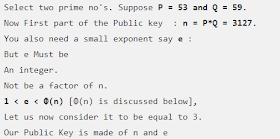## Recent PostsCryptography is a technique of encrypting clear plain text into a jumbled code. This encrypted data is know as "Ciphertext". This encrypted data is dencrypted by recipients by the same private or public key through which the data was encrypted.Cryptography is used to protect confidential data such as email messages, chat sessions, web transactions, personal data, corporate data, e-commerce applications, etc. Cryptography not only protects data from theft or alteration, but can also be used for user authentication. Cryptography is based on mathematical formula and structured algorithms

Types of Cryptography.

1.Symmetric Encryption Cryptography

Symmetric encryption cryptography is a method in cryptography where the same key is used for encryption as well as for  decryption. This key can be shared within multiple users for accessing the file.
This keys are made with different kinds of encryption algorithms.

Some common algorithm for symmetric encryption cryptography are:

Data Encryption Standard (DES)- It uses the 56 bit encryption algorithm.Advanced Encryption Standard (AES)- It uses the 128 bit encryption algorithm.Symmetric Encryption Cryptography

2.Asymmetric Encryption Cryptography

Asymmetric Encryption Cryptography is a method in cryptography where different keys are used for encryption and decryption. These keys are called as Public and Private keys. A public key is made freely available to anyone who might want to send you a message. The second private key is kept a secret so that you can only know.

A message that is encrypted using a public key can only be decrypted using a private key, while also, a message encrypted using a private key can be decrypted using a public key. Security of the public key is not required because it is publicly available and can be passed over the Internet

This keys are made with different kinds of encryption algorithms.

Some common algorithm for symmetric encryption cryptography are:

RSA AlgorithmDSA AlgorithmAsymmetric Encryption Cryptography

Algorithm in Cryptography

An algorithm is basically a set of commands or formula to solve any data snooping problem.  An encryption algorithm in Cryptography is a set of mathematical procedure for performing encryption on data. Through this algorithms data is converted into ciphers (crypted data)

Types Of  Encrypting Algorithm in Cryptography

1.Data Encryption Algorithm (DES)

The algorithm is designed to Encrypt and decrypt blocks of data of 64-bit under control of a 56 bit key.

DES is the archetypal block cipher- an algorithm that takes a fixed length string of plain text bits and transform it into a encrypted text.

Due to the inherent weakness of DES with today's technologies, some organization repeat the process three time (3DES) for added strength, until they can afford to update their equipment to AES
capabilities.Data Encryption Standard Algorithm

2. Advanced Encryption Standard (AES)

Advanced Encryption Standard (AES) is a symmetric-key algorithm for securing sensitive but unclassified material by US government agencies.

Advanced Encryption Standard is an iterated block cipher, which works by repeating the same operation multiple times.

It has a 128-bit block size, with key sizes of 128,192 and 256 bit.Advanced encryption standard

3. Rivest Shamir Adleman (RSA)

Rivest Shamir Adleman (RSA) is an Internet encryption and authentication system that uses an algorithm developed by Ron Rivest , Adi Shamir and Leonard Adleman.

Rivest Shamir Adleman (RSA) encryption is widely used and is one of the defector encryption standard.

Rivest Shamir Adleman (RSA) uses modular arithmetic and elementary number theories to perform computation using two large prime numbers.Rivest Shamir Adleman (RSA)

Hashing

Hashing is generating a value or values from a string of text using a mathematical function.

Hashing is one way to enable security during the process of message transmission when the message is intended for a particular recipient only. An Algorithm generates the hash, which helps to protect the security of the transmission against tampering.
Hashing is a one way function. A hash cannot be reversed to its original form.

Most of the Hackers uses Rainbow table ( A rainbow table is a precomputed table for reversing cryptographic hash functions ) to match their hashes to any predefined hash.

Types of Hashes.

1. Message Digest Function ( MD5)

Message Digest Function ( MD5) is a hashing algorithm that takes an input of any length and returns an output of 128-bit encrypted data.

It is used in digital signatures, file integrity checking and storing passwords .

Example:

Normal text : Hello

MD5 hash    : 8b1a9953c4611296a827abf8c47804d7MD5 hashing

2. Secure Hashing Algorithm (SHA)

Secure Hashing Algorithm (SHA) is another famous and secure Hashing algorithm used on a large scale. Secure Hashing Algorithm SHA-1 is a secure hashing algorithm producing 160-bit hashing value as compared ti MD5 produced 128-bit value. However SHA-2 is even more secure and safer hashing algorithm  now.

Example:

Normal text : Hello

SHA 1 hash : f7ff9e8b7bb2e09b70935a5d785e0cc5d9d0abf0Secure Hashing Algorithm SHA-1

Code Breaking Methodologies

Code Breaking Methodologies includes several tricks and techniques such as through social engineering techniques which are helpful to break  encryption and expose the information it it like cryptographic keys and messages.
These are some effective techniques and methodologies:

Brute-forceOne-Time padFrequency analysis Rainbow Tables

Cryptography - The Art of Encrypting DataReviewed by Shirley Bloggastron on 10:04 AM Rating: 5